• ## Statistics in the Triad, Part II: Log-Ratio Transformation

This post is a continuation of Statistics in the Triad, Part I: Geometric Mean. The two are meant to be read sequentially, since the mathematical elements of the first are an important and inescapable prerequisite for the second. If you already have a working knowledge of the geometric mean, however, and how its use differs from that of the ubiquitous arithmetic mean, then you can just read on.

### The closure constraint

The fundamental property of a triad or ternary plot that requires special consideration when applying statistics is its constant-sum or closure constraint. In a SenseMaker project, the data automatically sum to 100% when a respondent clicks or places a marker inside the triad on a collector screen; or the results are normalized (see Part I) if the respondent otherwise enters numerical values for each of the three components (vertices), which are then divided by the sum of the three values to yield percentages.

The constraint arises because, once the normalization step is completed, only two of the three variables are independent. If you think of the original data as being plotted in three-dimensional Cartesian coordinates, then the triad is essentially a projection from three to two dimensions:

This results in a loss of one degree of freedom, which means that if one of the variables is changed, the combination of the other two must necessarily change as well, but in the opposite direction. Thus, spurious negative correlations can arise among the data that fall completely outside any interpretive, subject-matter context, such as a SenseMaker project. This problem has been recognized for more than a century (Pearson, 1897), though it did not gain widespread notice until geologists began looking for workarounds in the 1960s and 1970s, prompted by a paper by Chayes (1960). In addition, because the bounded compositional data (in the range 0 to 1) of a triad cannot have a normal distribution, any statistical method that assumes non-bounded data (typically -∞ to +∞) cannot be used, for example, factor and principal component analysis. Even the lowly arithmetic mean is suspect.

A “practical tool of analysis” came in the 1980s in a series of papers by the statistician John Aitchison, fully articulated in his 1986 book (p. 112, reprinted in 2003 with some supplementary materials): The Statistical Analysis of Compositional Data. In the subsequent three decades, there has been considerable work on clarifying and extending the theoretical underpinnings of Aitchison’s work, in particular by Vera Pawlowsky-Glahn, Juan-José Egozcue, and their collaborators. The most succinct summary of these results can be found in their 2006 paper (see References); and a more extended treatment is given in their 2015 book, Modeling and Analysis of Compositional Data. Several others among the Additional Readings are less accessible and not for the mathematically-faint-of-heart.

Aitchison pulled that old rabbit, the coordinate transformation, out of the mathematician’s hat. Think Cartesian-to-polar coordinates or one of the other transformations used in the applied world when a difficult problem in one geometric setting magically becomes straightforward in another. (If you dig into the Additional Readings, you will find that each of the three log-ratio transformations now in use has some drawback in one or more coordinate systems. We will focus on only one of the three, however, and will ignore those issues for the sake of clarity, or reduced obscurity if you prefer.)

Here’s the setup for data point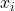in a triad displaying components,,:

(1)which applies for each of thedata points that may be available for that triad.

Aitchison then introduced the (additive) log-ratio transformation, which changes (1) to this:

(2)Schematically, the transformation looks like this:At a stroke, this creates two independent variables and moves the data from the closed ternary space (the “simplex”) to the domain of real numbers (-∞ to +∞), thereby eliminating spurious negative correlations and other covariance problems and opening up the transformed data to a variety of standard statistical methods. Equally important, there is an inverse transformation that allows results to be brought back to the triad for display with the original data.

Here is a concrete example, reconstructed and modified slightly from Aitchison (1989, Fig. 1; 1986, Table 1.2), to illustrate the procedure: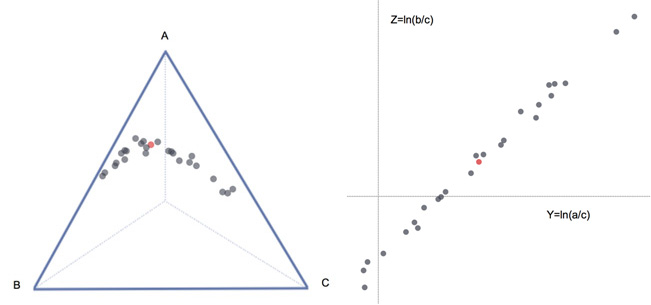The triad shows data points for 25 samples of the rock type “hongite.” (This is one of Aitchison’s droll inventions, along with “kongite,” “coxite,” and “boxite,” presumably based on his time at the University of Hong Kong and the statisticians David Cox and George Box, respectively.) Each data point satisfies (1); the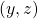plot shows those same samples after transformation by (2). Because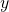and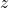are independent, the arithmetic mean of each can be calculated from these relations:

(3)Notice that (3) should be compared to the left-hand equation forin (5) of Part I, where the terms being summed are now of the form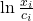.

The arithmetic mean calculated from (3) for the transformed data is shown as the red dot in the right-hand graph above. Not surprisingly, it falls “midway” along the distribution. The red dot is also shown in the triad. We’ll look now at how that return step, the inverse transformation, was done and the significance of the resulting point.

### Back to the triad: the inverse transformation

The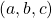coordinates in the triad for the arithmetic meanin the log-ratio plot can be calculated from the inverse transformation, which exponentiates the independent terms and normalizes them back to a constant sum of 1 in:

(4)(5)(6)The appearance ofas the third term in the denominator of each equation, and as the numerator in (6), literally creates the closure (constant sum) required for the ternary plot. (The reason for the change in notation on these coordinates — the overset bar to denote mean and the subscript to connect back to the log-ratio plot — will become clear as we proceed.)

Each of (4)-(6) can be expanded by substituting (3), but we will only do this for (4) in the interest of brevity. The other two will look similar to this:

(7)Now we can begin to simplify and make a surprising discovery. Using standard logarithmic identities, including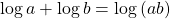, we can collapse each of the summations to a product:

(8)This right-hand expression in (8) should look familiar (see (12) in Part I) — it is the geometric mean of the ratiofor thedata points.

We can now substitute (8) into (7) for each of the summation terms, and by implication do the same for each of equations (4)-(6):

(9)If we make the trivial substitution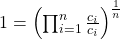in the denominator of (9), then the common factor,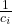, will cancel in all four terms, top and bottom, leaving this:

(10)The numerator in (10) is by definition the geometric mean of the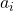component for the data points in the triad; and the denominator normalizes it to the sum of the geometric means for all three components. Similarly for the other two components,and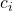. Thus, the arithmetic mean as calculated with (3) in the log-ratio plot — the red dot — inverse-transforms to thecoordinates in the triad — also the red dot. Surprising, confusing, unsettling… but true! As Aitchison (1989, p. 789) says, this is precisely “the composition formed from [the] geometric means by the process of closure” (Aitchison, 1989, p. 789). In other words, the arithmetic mean in the log-ratio plot is identical to the geometric mean in a triad.

As a practical matter, if we only wanted to know the geometric mean and had no interest in other statistical calculations with the log-ratio data, then we could skip the transformation entirely and simply calculate the geometric mean directly (see (5) or (12) in Part I). Red dots all around, and far fewer of those big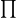s and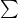s.

### Array shapes in the triad

We can both visualize this relationship and understand its significance by re-visiting the ternary plot for Aitchison’s “hongite” compositions: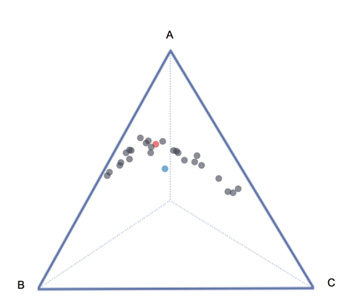To emphasize, the red dot is the inverse transformation of the arithmetic mean in the log-ratio plot, but as we have just seen it is really the geometric mean in the triad. By contrast, the blue dot is Aitchison’s (1989, p. 329) composition of the arithmetic mean of each of the three individual,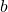, andcomponents in the triad. He succinctly sums up the problem with the blue dot, the arithmetic mean (emphasis added): “It is clearly useless as a measure of location because it falls outside the array of compositions and is indeed very atypical of the data set.”

Although the distinction between the arithmetic and geometric means is enhanced visually when the data are in a well-defined, curved array like this, the general principle remains: the geometric mean is the appropriate “measure of location” for constant-sum data. What if the data are just an amorphous blob? It doesn’t matter — use the geometric mean! OK, but what if the data look more like a typical SenseMaker study, with respondents’ dots located near the center, near the three vertices, and along the three bisectors? It doesn’t matter — use the geometric mean, whether for the entire data set or for cohort- or signifier-defined subsets.

There is one situation, however, where careful inspection of and experimentation with data patterns might be particularly helpful. Some of the curved arrays encountered in geochemical studies of volcanic rocks have a temperature-dependence, displaying changing composition as lavas and magmas cool and crystallize. Under the broad rubric of thermodynamics, “cooling” means “passing time.” So moving “down” such a data array would, in general, be tracking time. That thought evokes SenseMaker projects employing either punctuated or continuous capture of data, where a fixed group of prompts and signifiers is used over an extended period of time. In that situation, especially if various interventions occurred in the hope of eliciting a change in behavior/response, then tracking time would be both necessary and valuable. A study that was well-designed in this regard might be the perfect way to persuade a skeptic — for example, a client who didn’t fully appreciate the wonders of the math — that the geometric mean is the right “measure of location” to use. And once demonstrated to the skeptic’s satisfaction, the point would carry over to any distinctions among data, whether temporal or demographic or cultural.

### References

Aitchison, J. (1986, reprinted 2003) The Statistical Analysis of Compositional Data. The Blackburn Press, Caldwell NJ. 416 pp. plus additional material.

Aitchison, J. (1989) Measures of Location of Compositional Data Sets. Mathematical Geology, v. 21, p. 787-790.

Chayes, F. (1960) On correlation between variables of constant sum. Journal of Geophysical Research, v. 65, p. 4185-4193.

Pawlowsky-Glahn, V., and Egozcue, J.J. (2006) Compositional data and their analysis: an introduction. in Buccianti, A., Mateu-Figueras, G., and Pawlowsky-Glahn, V., editors, Compositional Data Analysis in the Geosciences: From Theory to Practice, Geological Society of London, Special Publications 264, p. 1-10.

Pearson, K. (1897) Mathematical contributions to the theory of evolution. On a form of spurious correlation which may arise when indices are used in the measurement of organs. Proceedings of the Royal Society of London, v. 60, p. 489-502.

Egozcue, J.J., and Pawlowsky-Glahn, V. (2005) Groups of parts and their balances in compositional data analysis. Mathematical Geology, v. 37, p. 795-828.

Egozcue, J.J., and Pawlowsky-Glahn, V. (2006) Simplicial geometry for compositional data. in Buccianti, A., Mateu-Figueras, G., and Pawlowsky-Glahn, V., editors, Compositional Data Analysis in the Geosciences: From Theory to Practice, Geological Society of London, Special Publications 264, p. 145-159.

Egozcue, J.J., Pawlowsky-Glahn, V., Mateu-Figueras, G., and Barcelo-Vidal, C. (2003) Isometric logratio transformation for compositional data analysis. Mathematical Geology, v. 35, p. 279-300.

Pawlowsky-Glahn, V., Egozcue, J.J., and Tolosana-Delgado, R. (2015) Modeling and Analysis of Compositional Data. John Wiley & Sons, New York. 272 pp.

van den Boogaart, K.G., and Tolosana-Delgado, R. (2013) Analyzing Compositional Data with R. Springer-Verlag, Berlin. 258 pp.

von Eynatten, H., Pawlowsky-Glahn, V., and Egozcue, J.J. (2002) Understanding perturbation on the simplex: a simple method to better visualise and interpret compositional data in ternary diagrams. Mathematical Geology, v. 34, p. 249-257.